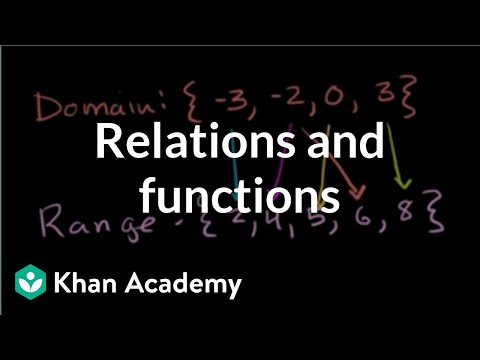## Relation

Subject: Optional Mathematics#### Overview

A relation between two sets is a collection of ordered pairs containing one object from each set. If the object x is from the first set and the object y is from the second set, then the objects are said to be related if the ordered pair (x,y) is in the relation. A technical definition of a function is a relation from a set of inputs to a set of possible outputs where each input is related to exactly one output. This means that if the object x is in the set of inputs (called the domain) then a function fh

##### RelationLet A and B be two sets. Then, A×B is called the Cartesian product of A and B. Any subset of A×B is called a relation from A to B. A relation from A to B is denoted by R:A→B or simply by R. For example,

Let A = {1,2} and B = {3,4}

Then, AB = {(1,3),(1,4),(2,3),(2,4)}

Let, R = {(1,3),(2,3)}

Here, R is a subset of A×B. So, R is a relation in A.

#### Domain and Range of the Relation

A relation from set A to set B is defined by a subset of Cartesian product A× B under the certain condition. The relation is generally denoted by R: A → B.

The first set A is called Domain of relation.

Second set B is called range of the relation.

#### Representation of relation

Relation may be expressed in various ways. Some ways are:a. Listing or ordered pair form
R= {(Hari,Sujit)(Uma,Sharmila)(Sagarmatha,Dhaulagiri)}
R = {(1,1),(2,2),(3,3)}

b. Table form
Let, A = {1,2,3} and B = {1,4,3}.
Define R = {(x,y):y=x2}
Then, the relation R can be represented as:

 x 1 2 3 y 1 4 9

c. Set builder form
Let A = {1,2,3} and B = {4,5,6} , Define R = {(x,y):x=y} i.e. R= {(1,1),(2,2),(3,3)}

d. Graphical form
Let A = {1,2,3} and B = {1,2,3}, R={(x,y):y=x2}

e. Arrow diagram form
Let A = {a, b} and B = {1, 2}

### Inverse of a relation

If R = {(a,b): a∈A and b∈B} is a relation, then there exists another relation {(b, a) b∈B and a∈A} which is known as the inverse relation of R.

it is denoted by R-1.

Mathematically, R-1= [(b,a) : b∈ B and A ∈ a}

For example, If R = {( 1,a), (2,b), (3,c)}, then

R-1= {(a,1), (b,2), (c,3)}

##### Things to remember
• Any subset of A×B is called a relation from A to B.
• A relation from set A to set B is defined by a subset of Cartesian product A× B under the certain condition.
• The first set A is called Domain of relation. Second set B is called range of the relation.

• It includes every relationship which established among the people.
• There can be more than one community in a society. Community smaller than society.
• It is a network of social relationships which cannot see or touched.
• common interests and common objectives are not necessary for society.
##### Videos for Relation##### Relations and functions | Functions and their graphs | Algebra II | Khan Academy

soln:

here set of younger brother ={Laxman,Arjun,Dhirendra}

Set of elder brother= {Ram,Bhimsen,Pravin}

The mapping diagram to show the relationship from younger to elder brother is as shown below:

∴ Relation of younger and Elder brother = {(Laxman,Ram), (Arjun,Bhimsen), (Dhirendra, Pravin)} Ans.

Soln:

Here given sets are:

A= {4,2,36,24}, B={1,2,18,12}

The mapping diagram from A to B show the relationship "Double of" is as shown below:

∴ "Double of " relation= {(4,2),(2,1)(36,18),(24,12)} Ans.

Soln:

here given,

Set of persons = { Ranju,Anju,Bharat,Radha}

Set of characteristics = {tall,black,short}

The mapping diagram from set of persons to set of characteristics is as shown below:

From the above mapping diagram:

(a) Ranju is tall and black

(b) Bharat is short and black

(c) Anju is tall and Radha is short. Ans.

Soln:

From figure in Q.9 (b)

(a) Since 2 and 3 both are related to 1, so 2 and 3 have a common factor 1. Ans.

(b)Since 1 is related to 1 only. So, common factor of 1 is 1. Ans.

(c) Since 2 is related to 1 and 2. So the common factors of 2 are 2 and 1. Ans.

soln:

here given,

A={3,4,5,6,7} and B={1,2,3,4}

The mapping diagram from A to B to show the relationship 'less than 2' is as shown below:

Here each number of B is less than 2 then the number from A.

∴ Relation= {(3,1),(4,2),(5,3),(6,4). Ans

Soln:

here given,

Now,

Soln:

(a) Here given arrow diagram:

Relation between P and Q={(1,1),(2,4)(3,9)} which is the square relation.Ans

(b) Here given arrow diagram:

Relation between P and Q= {(1,1),(1,2),(1,3),(2,2),(3,3)} which is the less or equal to relation. Ans.

Soln:
∴ A×B= {(3,4),(3,5),(3,6),(3,7),(4,4),(4,5),(4,6),(4,7),(5,4),(5,5),(5,6),(5,7),(6,4),(6,5),(6,6),(6,7)}. Ans.

The mapping diagram of A×B is given below:

∴ A×B={(3,4),(3,5),(3,6),(3,7),(4,4),(4,5),(4,6),(4,7),(5,4),(5,5),(5,6),(5,7),(6,4),(6,5),(6,6),(6,7)}. Ans.

The graph of A×B is given below:

Soln:

The arrow diagram From A to B to show relationship "is the capital of " is as follow: